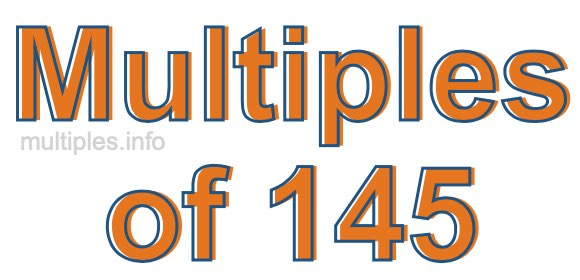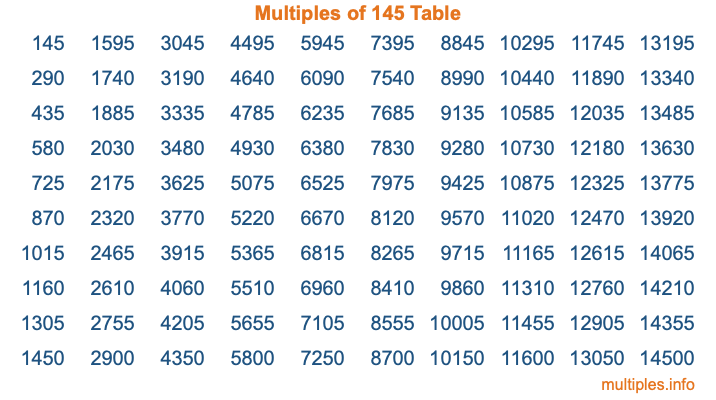Multiples of 145Welcome to the Multiples of 145 page. Here we will first teach you everything you will ever need to know about the multiples of 145, and then give you a study guide summary of everything we taught you to make sure you remember it all. Use this page to look up facts and learn information about the multiples of 145. This page will make you a multiples of one hundred forty-five expert!

Definition of Multiples of 145
Multiples of 145 are all the numbers that when divided by 145 equal an integer. Each of the multiples of 145 are called a multiple. A multiple of 145 is created by multiplying 145 by an integer.

Therefore, to create a list of multiples of 145, you start with 1 multiplied by 145, then 2 multiplied by 145, then 3 multiplied by 145, and so on for as long as you want. Thus, the list of the first five multiples of 145 is 145, 290, 435, 580, and 725. To see a larger list of multiples of 145, see the printable image of Multiples of 145 further down on this page. We also have a category where you can choose any nth multiple of 145.

Multiples of 145 Checker
The Multiples of 145 Checker below checks to see if any number of your choice is a multiple of 145. In other words, it checks to see if there is any number (integer) that when multiplied by 145 will equal your number. To do that, we divide your number by 145. If the the quotient is an integer, then your number is a multiple of 145.

Is  a multiple of 145?

Least Common Multiple of 145 and ...
A Least Common Multiple (LCM) is the lowest multiple that two or more numbers have in common. This is also called the smallest common multiple or lowest common multiple and is useful to know when you are adding our subtracting fractions. Enter one or more numbers below (145 is already entered) to find the LCM.

Check out our LCM Calculator if you need more details about the Least Common Multiple or if you need the LCM for different numbers for adding and subtraction fractions.

nth Multiple of 145
As we stated above, 145 is the first multiple of 145, 290 is the second multiple of 145, 435 is the third multiple of 145, and so on. Enter a number below to find the nth multiple of 145.

th multiple of 145

Multiples of 145 vs Factors of 145
145 is a multiple of 145 and a factor of 145, but that is where the similarities end. All postive multiples of 145 are 145 or greater than 145. All positive factors of 145 are 145 or less than 145.

Below is the beginning list of multiples of 145 and the factors of 145 so you can compare:

Multiples of 145: 145, 290, 435, 580, 725, etc.

Factors of 145: 1, 5, 29, 145

As you can see, the multiples of 145 are all the numbers that you can divide by 145 to get a whole number. The factors of 145, on the other hand, are all the whole numbers that you can multiply by another whole number to get 145.

It's also interesting to note that if a number (x) is a factor of 145, then 145 will also be a multiple of that number (x).

Multiples of 145 vs Divisors of 145
The divisors of 145 are all the integers that 145 can be divided by evenly. Below is a list of the divisors of 145.

Divisors of 145: 1, 5, 29, 145

The interesting thing to note here is that if you take any multiple of 145 and divide it by a divisor of 145, you will see that the quotient is an integer.

Multiples of 145 Table
Below is an image of the first 100 multiples of 145 in a table. The table is in chronological order, column by column. The first column has the first ten multiples of 145, the second column has the next ten multiples of 145, and so on.The Multiples of 145 Table is also referred to as the 145 Times Table or Times Table of 145. You are welcome to print out our table for your studies.

Negative Multiples of 145
Although not often discussed or needed in math, it is worth mentioning that you can make a list of negative multiples of 145 by multiplying 145 by -1, then by -2, then by -3, and so on, to get the following list of negative multiples of 145:

-145, -290, -435, -580, -725, etc.

Multiples of 145 Summary
Below is a summary of important Multiples of 145 facts that we have discussed on this page. To retain the knowledge on this page, we recommend that you read through the summary and explain to yourself or a study partner why they hold true.

There are an infinite number of multiples of 145.

A multiple of 145 divided by 145 will equal a whole number.

145 divided by a factor of 145 equals a divisor of 145.

The nth multiple of 145 is n times 145.

The largest factor of 145 is equal to the first positive multiple of 145.

145 is a multiple of every factor of 145.

145 is a multiple of 145.

A multiple of 145 divided by a divisor of 145 equals an integer.

145 divided by a divisor of 145 equals a factor of 145.

Any integer times 145 will equal a multiple of 145.

Multiples of a Number
Here you can get the multiples of another number, all with the same attention to detail as we did for multiples of 145 on this page.

Multiples of
Multiples of 146
Did you find our page about multiples of one hundred forty-five educational? Do you want more knowledge? Check out the multiples of the next number on our list!

Copyright  |   Privacy Policy  |   Disclaimer  |   Contact